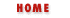### 8.6 Array Methods

In the previous section we saw that--in Navigator 3.0 and Internet Explorer 3.0--arrays created with the Array() constructor have a length property. In Navigator 3.0, but not in IE 3.0, these arrays also support three methods that can be used to manipulate the array elements. These methods will be implemented in a future version of IE.

The Array.join() method converts all the elements of the array to a string, and concatenates them, separating them with an optionally specified string passed as an argument to the method. If no separator string is specified, then a comma is used. For example, the following lines of code produce the string "1,2,3":

```a = new Array(1,2,3);  // Create a new array with these three elements.
s = a.join();          // s == "1,2,3"
```
And the following lines specify the optional separator to produce a slightly different result:

```a = new Array(1,2,3);
s = a.join(", ");   // s == "1, 2, 3". Note the space after the comma.
```
In some ways, the Array.join() method is the reverse of the String.split() method which creates an array by breaking a string up into pieces.

The Array.reverse() method reverses the order of the elements of an array. It does this "in place"--i.e., it doesn't create a new array with the elements rearranged, but instead rearranges them in the already existing array. For example, the following code, which uses the reverse() and the join() methods, produces the string "3,2,1":

```a = new Array(1,2,3);   // a = 1; a = 2; a = 3;
a.reverse();            // now a = 3; a = 2; a = 1;
s = a.join()            // s = "3,2,1"
```

The final array method is Array.sort(), which sorts the elements of an array. Like the reverse() method, it does this "in place". When sort() is called with no arguments, it sorts the array elements in alphabetical order (temporarily converting them to strings, to perform the comparison, if necessary):

```a = new Array("banana", "cherry", "apple");
a.sort();
s = a.join(", ");   // s == "apple, banana, cherry".
```
You can also pass an argument to the sort() method if you want to sort the array elements in some other order. To allow this method to be a fully general sorting algorithm, the optional argument should be a function. This function will be passed two arguments that it should compare. If the first argument should appear before the second in the sorted array, then the function should return a number less than zero. If the first argument should appear after the second in the sorted array, then the function should return a number greater than zero. And if the two values are equivalent (their order is irrelevant), then the function should return 0. So, for example, to sort array elements into numerical, rather than alphabetical order, you might do the following:

```a = new Array(33, 4, 1111, 222);
a.sort();                        // alphabetical order:  1111, 222, 33, 4
function numberorder(a,b) {
return a-b;
}
a.sort(numberorder);             // numerical order: 4, 33, 222, 1111
```
You can probably think of other comparison functions that will sort numbers into various esoteric orders: reverse numerical order, odd numbers before even numbers, etc. The possibilities become more interesting, of course, when the elements you are comparing are objects rather than simple types like numbers or strings.Array Length PropertyArrays in Navigator 2.0